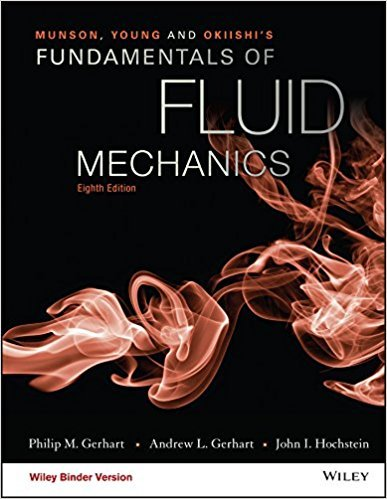×
Get Full Access to Fundamentals Of Fluid Mechanics - 8 Edition - Chapter 5.1.2 - Problem 5.4
Get Full Access to Fundamentals Of Fluid Mechanics - 8 Edition - Chapter 5.1.2 - Problem 5.4

×

# Water flows out through a set of thin, closely spaced blades asshown in Fig. P5.4 with aISBN: 9781119080701 456

## Solution for problem 5.4 Chapter 5.1.2

Fundamentals of Fluid Mechanics | 8th Edition

• Textbook Solutions
• 2901 Step-by-step solutions solved by professors and subject experts
• Get 24/7 help from StudySoup virtual teaching assistantsFundamentals of Fluid Mechanics | 8th Edition

4 5 1 297 Reviews
30
0
Problem 5.4

Water flows out through a set of thin, closely spaced blades asshown in Fig. P5.4 with a speed of V = 10 ft/s around the entirecircumference of the outlet. Determine the mass flowrate throughthe inlet pipe.

Step-by-Step Solution:
Step 1 of 3

CHM 101 – CHAPTER 3 NOTES – STOICHIOMETRY Section 3.1 – 3.3 – Atomic Mass, Avogadro’s Number, Molar Mass, and Molecular Mass 23 Avogadro’s number: 6.022 * 10 particles = 1 mol particles; used to convert between atoms, molecules, and masses Subscripts give mole ratios of atoms in a compound. Atomic mass: mass of one atom of a substance in amu 1 amu = 1/12 of the mass of one C-12 atom Molar mass (MM): the mass of one mole of substance (atoms or molecules) Molecular mass: mass of one molecule of a substance in amu; sum of atomic masses (in amu) in a molecule Section 3.7 – Chemical Reactions and Chemical Equations Reactants yield Products Rules of balancing chemical equations: 1. If an element is present in just 1 compound on each side of the equation, balance that element first 2. Balance free elements last 3. Fractions can be cleared at any time by multiplying all coefficients by a common multiplier 4. Groupings of atoms may remain unchanged; balance them as a group Section 3.8 – Stoichiometry: Amounts of Reactants and Produts Reactions rarely yield maximum product Actual Yield: yield recovered during the experiment Theoretical Yield: yield calculated from limiting reagent; maximum obtainable yield Percent yield = (actual yield)/(theoretical yield) * 100% Section 3.9 – 3.10 – Limiting Reagents and Reaction Yield Limiting Reagent: reactant that runs out first; find by calculating the moles of product from each given amount of reactant; reactant producing the least amount of product Excess reagent: re

Step 2 of 3

Step 3 of 3

##### ISBN: 9781119080701

Unlock Textbook Solution